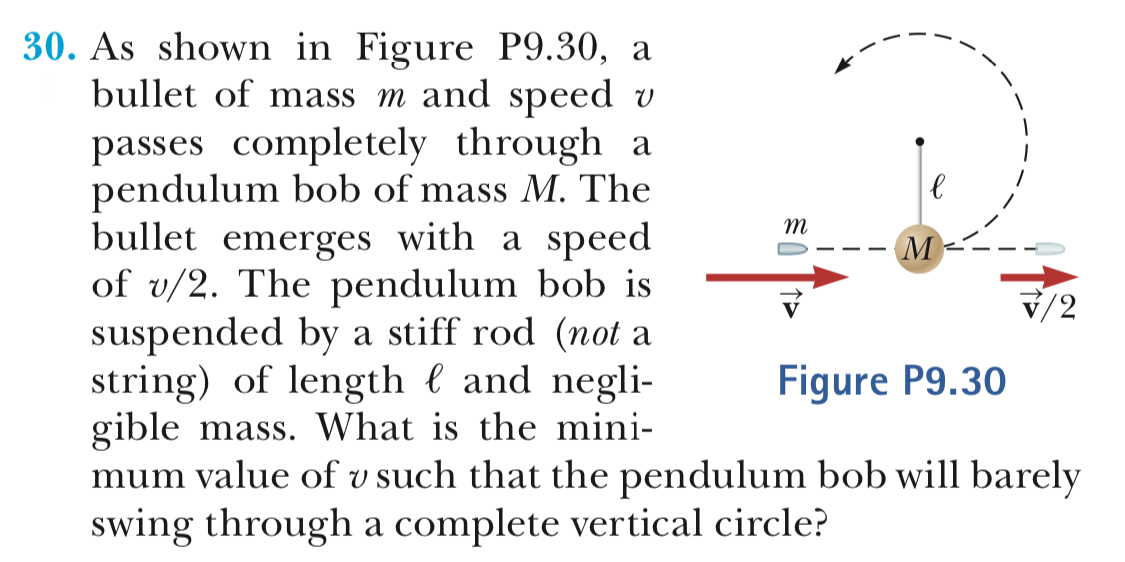30. As shown in Figure P9.30, abullet of mass m and speed vpasses completely through apendulum bob of mass M. Thebullet emerges with a speedof v/2. The pendulum bob issuspended by a stiff rod (not astring) of length l and negli-giblemass. What is the mini-V/2Figure P9.30mum value of v such that the pendulum bob will barelyswing through a complete vertical circle?

Questionhelp_outlineImage Transcriptionclose30. As shown in Figure P9.30, a bullet of mass m and speed v passes completely through a pendulum bob of mass M. The bullet emerges with a speed of v/2. The pendulum bob is suspended by a stiff rod (not a string) of length l and negli- giblemass. What is the mini- V/2 Figure P9.30 mum value of v such that the pendulum bob will barely swing through a complete vertical circle? fullscreen
Step 1

Given that the mass of the bullet is m, its speed before collision is v, the mass of the bob is M, the speed of the bullet after collision is v/2. The radius of the circular path of motion of the bob is l.

The pendulum initially stood still before the bullet was fired, and hence the initial speed of the bob is zero. Apply the conservation of linear momentum to the given system.

Step 2

Consider the initial position of the bob as zero level. So that the bob makes a maximum height equal to twice the length of the string. Consider two points. Point 1 at the ground level in which bob is initially located, and point 2 at the maximum height point which is given by 2l. Now apply the conservation of mechanical energy to the bob. The kinetic energy at the top of the vertical circle (point 2) is zero since the bob comes to rest momentarily there. The gravitational potential energy at the bottom of the circle is zero.

Step 3

Use equation (3) in (2) to find the minimum speed v such that the pendul...

Want to see the full answer?

See Solution

Want to see this answer and more?

Our solutions are written by experts, many with advanced degrees, and available 24/7

See Solution
Tagged in

Kinematics